# Mathematics Support

Math 8 | Unit 1 2 3 4 5 6 7 8 9

### Pythagorean Theorem and Irrational Numbers

The Size of Shapes

The Pythagorean Theorem

Side Lengths and Volumes of Cubes

## The Size of Shapes

This week your student will be working with the relationship between the side length and area of squares. We know two main ways to find the area of a square:

• Multiply the square’s side length by itself.
• Decompose and rearrange the square so that we can see how many square units are inside. For example, if we decompose and rearrange the tilted square in the diagram, we can see that its area is 10 square units.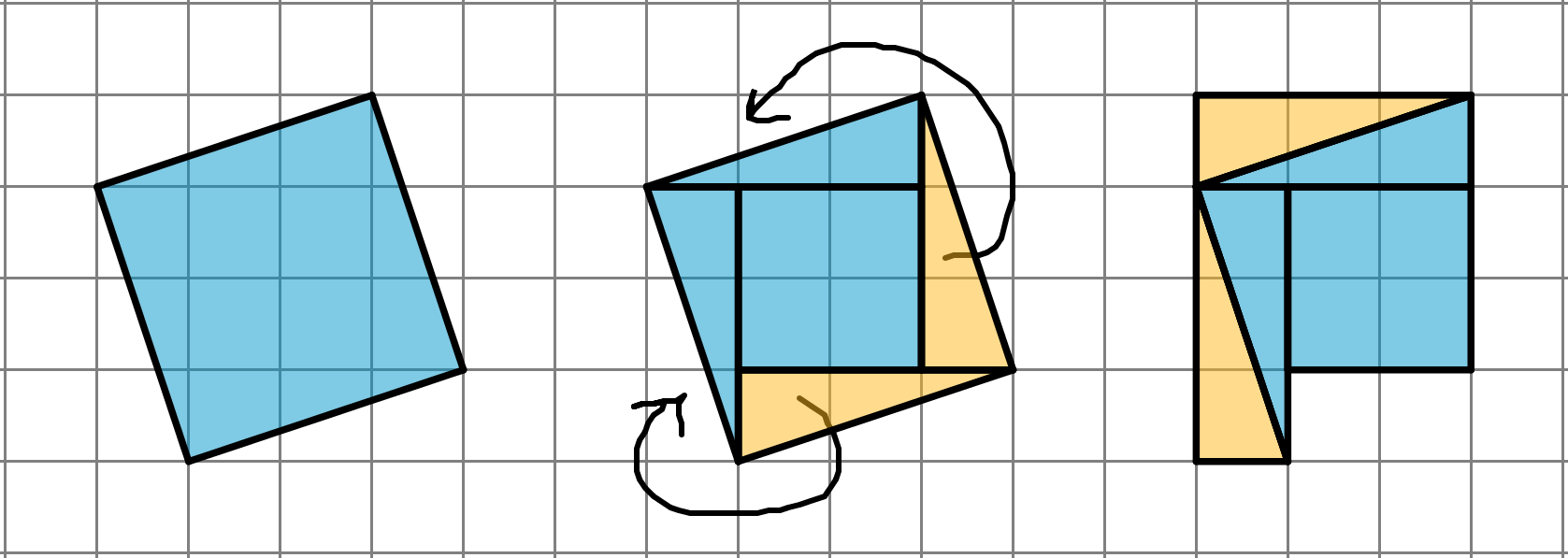But what is the side length of this tilted square? It cannot be 3 units since 32=9 and it cannot be 4 units since 42=16. In order to write “the side length of a square whose area is 10 square units,” we use notation called a square root. We write “the square root of 10” as 10 and it means “the length of a side of a square whose area is 10 square units.” All of these statements are true:

• 9=3 because 32=9
• 16=4 because 42=16
• 10 is the side length of a square whose area is 10 square units, and (10)2=10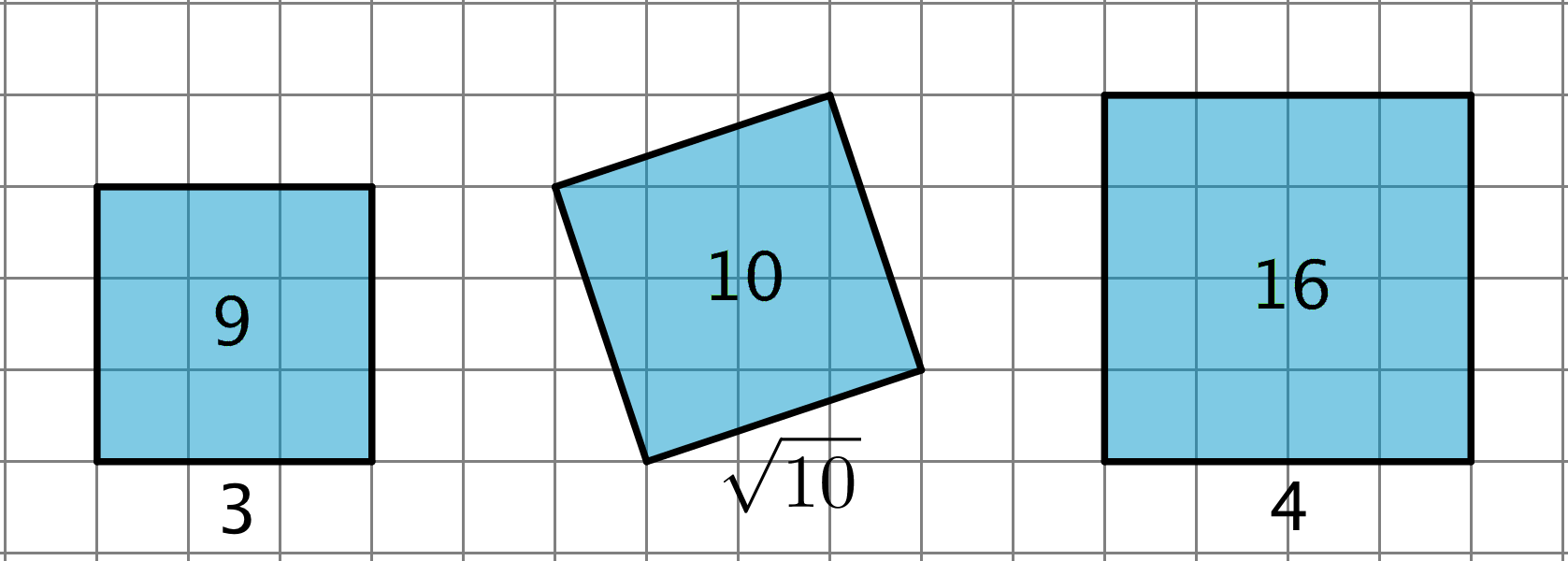If each grid square represents 1 square unit, what is the side length of this titled square? Explain your reasoning.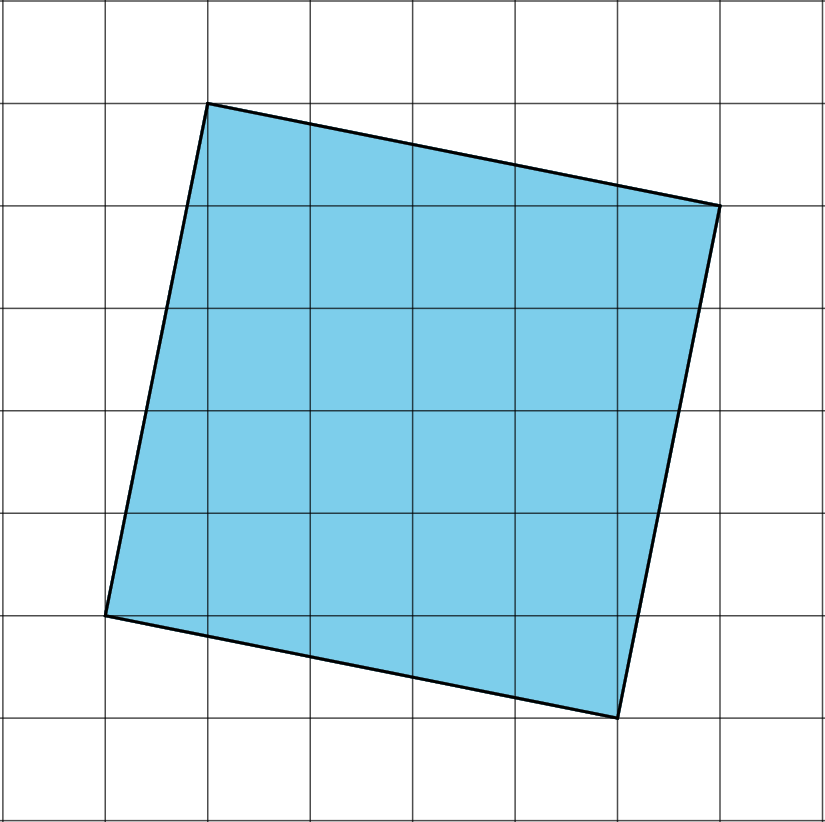Solution:

The side length is 26 because the area of the square is 26 square units and the square root of the area of a square is the side length.

## The Pythagorean Theorem

This week your student will work with the Pythagorean Theorem, which describes the relationship between the sides of any right triangle. A right triangle is any triangle with a right angle. The side opposite the right angle is called the hypotenuse, and the two other sides are called the legs. Here we have a triangle with hypotenuse c and legs a and b. The Pythagorean Theorem states that for any right triangle, the sum of the squares of the legs are equal to the square of the hypotenuse. In other words, a2+b2=c2.We can use the Pythagorean Theorem to tell if a triangle is a right triangle or not, to find the value of one side length of a right triangle if we know the other two, and to answer questions about situations that can be modeled with right triangles. For example, let’s say we wanted to find the length of this line segment: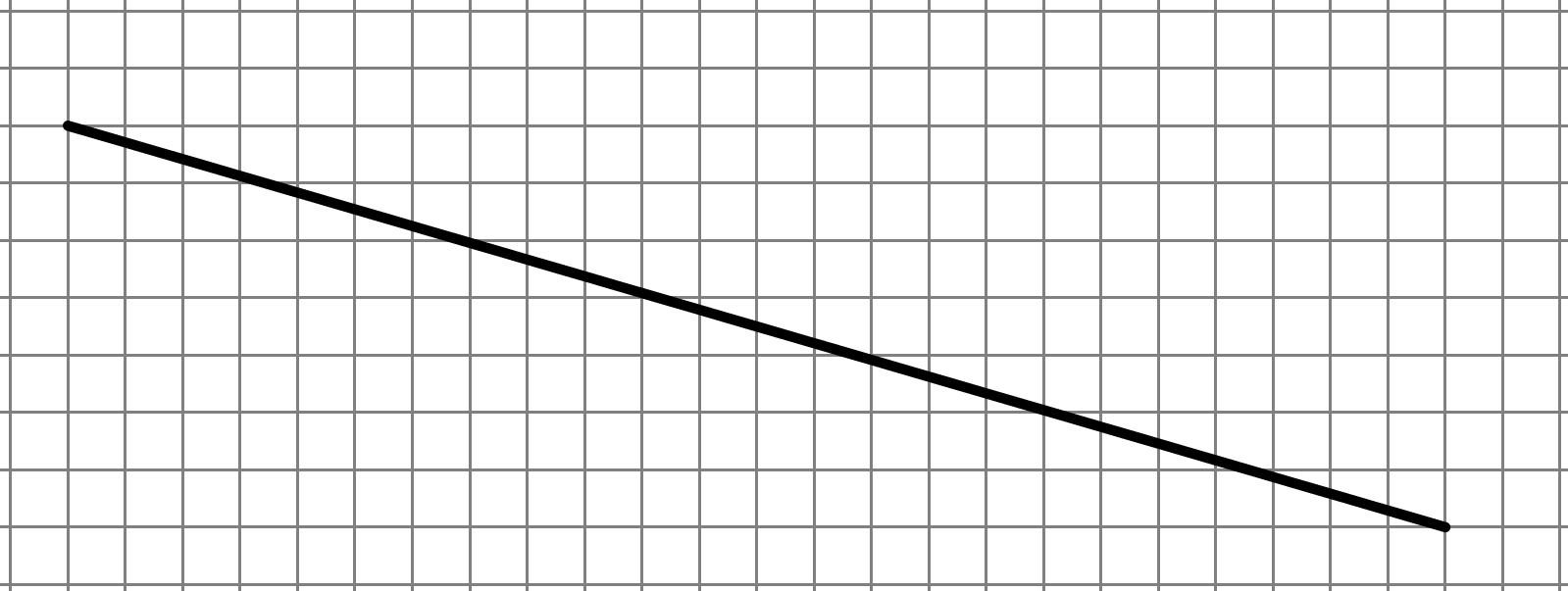We can first draw a right triangle and determine the lengths of the two legs: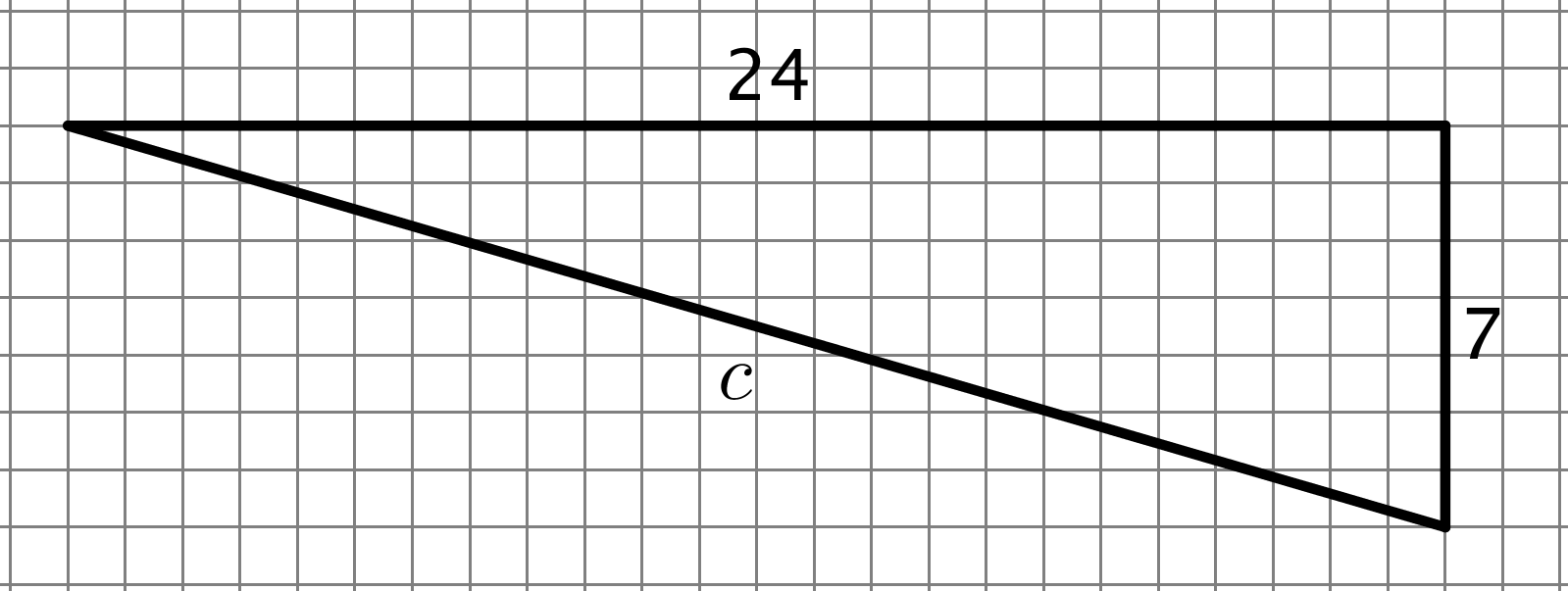Next, since this is a right triangle, we know that 242+72=c2, which means the length of the line segment is 25 units.

1. Find the length of the hypotenuse as an exact answer using a square root.2. What is the length of line segment p? Explain or show your reasoning. (Each grid square represents 1 square unit.)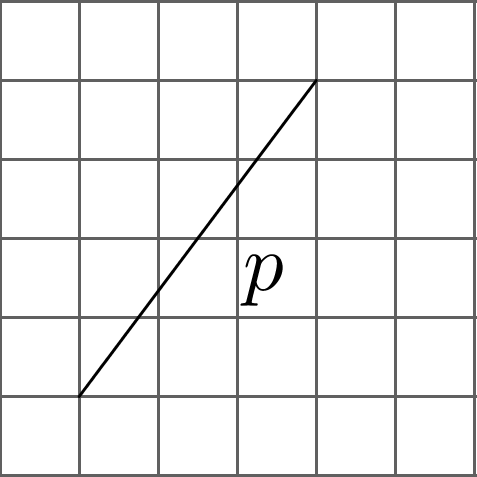Solution:

1. The length of the hypotenuse is 50 units. With legs a and b both equal to 5 and an unknown value for the hypotenuse, c, we know the relationship 52+52=c2 is true. That means 50=c2, so c must be 50units.
2. The length of p is 25 or 5 units. If we draw in the right triangle, we have legs of length 3 and 4 and hypotenuse p, so the relationship 32+42=p2 is true. Since 32+42=25=p2p must equal 25 or 5 units.

## Side Lengths and Volumes of Cubes

This week your student will learn about cube roots. We previously learned that a square root is the side length of a square with a certain area. For example, if a square has an area of 16 square units then its edge length is 4 units because 16=4. Now, think about a solid cube. The cube has a volume, and the edge length of the cube is called the cube root of its volume. In this diagram, the cube has a volume of 64 cubic units: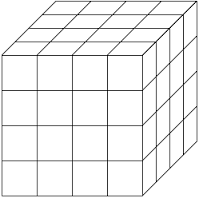Even without the useful grid, we can calculate that the edge length is 4 from the volume since 643=4.

Cube roots that are not integers are still numbers that we can plot on a number line. If we have the three numbers 40303, and 643, we can plot them on the number line by estimating what integers they are near. For example, 40 is between 6 and 7, since 36<40<49 and 36=6while 49=7. Similarly, 303 is between 3 and 4 because 30 is between 27 and 64. Our number line will look like this: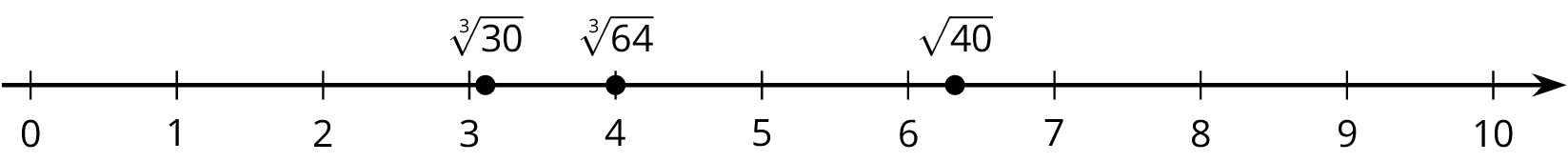Plot the given numbers on the number line: 28273503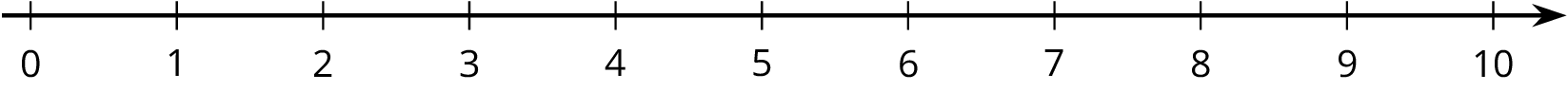Solution:

Since 33=27 means 273=3, we can plot 273 at 3. 503 is between 3 and 4 because 50 is between 33=27 and 43=6428 is between 5 and 6 because 28 is between 52=25 and 62=36.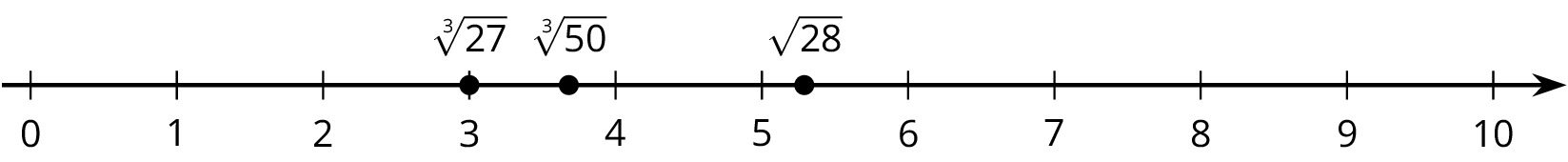IM 6–8 Math was originally developed by Open Up Resources and authored by Illustrative Mathematics, and is copyright 2017-2019 by Open Up Resources. It is licensed under the Creative Commons Attribution 4.0 International License (CC BY 4.0). OUR's 6–8 Math Curriculum is available at https://openupresources.org/math-curriculum/.

Disclaimer: This site provides external links and videos as a convenience and for informational purposes only. The appearance of external hyperlinks on the MCPS Family Mathematics Support Center website does not constitute an endorsement by the Montgomery County Public School System of any of the products or opinions contained therein.